## 小学五年级数学上册竞赛题

1.口算下面各题。（每题0.5分，共20分）

2.5-1.7= 0.28+0.2= 3.6+4.4= 0.38+5.52=

6.02×100= 10×4.62= 1.25×8= 100×4.62=

53÷100= 37.2÷10= 12x-7x= 224÷100=

1.8+0.2= 9+1.5= 1.9+0.5 = 0.65+0.35=

4.7+0.4= 9.3-4= 6.6+1.4= 5.6-4=

1.6÷2= 5.6÷7= 5.25÷5= 4.8÷3=

7.52-5= 7.2÷0.9= 12.3×0= 9.4×0.1=

35×0.2= 5a×a= 0.4×0.5= 0÷0.45=

0.8÷0.2= 1÷0.8= 2.4÷0.04= 7.5÷0.5=

3.5×0.5= 0.25×4= 0.8×0.06= 5b-4b=

2.列竖式计算。（每题2分，共8分。）

19-5.63= 7.58+3.24= 0.45×0.2= 5.25÷35=

3.计算下面各题。（每题3分，共12分。）

6.2+0.46+3.8+0.54 76.5-23.5-16.5+13.5

47.5×0.87+0.13×47.5= 0.25×0.125×32

1. 一个三位小数，四舍五入保留两位小数后是5.90，这个三位小数最大是（ ），最小是（ ）。
2. 用三张数字卡片1、2、3和小数点组成6个不同的两位小数，把它们按照从大到小的顺序排列出来 、 。
3. 一个三角形和一个平行四边形等底等高，三角形的面积是75平方厘米，它们们的面积和是（ ）平方厘米。
4. 服装厂运来450米布料，做90套儿童服装正好用去这批布的一半，平均每套服装用布（ ）米。
5. 已知A=0.00……060，B=0.00……03，则A÷B=（ ）。

2014个0

2014个0

6.在一次数学测验中，把高于平均分的部分记作正数。小丽得分是100分，记作+12分；小军得分记作-8分，小军的得分是（ ）分。

7.一个数除以m(m不为0)，商是8，余数是6，这个数是（ ）。

8.买3支圆珠笔和2支铅笔要8.7元，买2支圆珠笔和3支铅笔要6.8元。铅笔的单价是（ ）元，圆珠笔的单价是（ ）元。

9.甲乙两个数的和是16.5，甲数的小数点向右移动一位正好等于乙数。算一算，甲数是（ ），乙数是（ ）。

10.如图一个长方形被分成了一个一个三角形和一个梯形。已知三角形的面积比梯形少180平方厘米。三角形的面积是（ ）平方厘米，梯形的面积是（ ）平方厘米。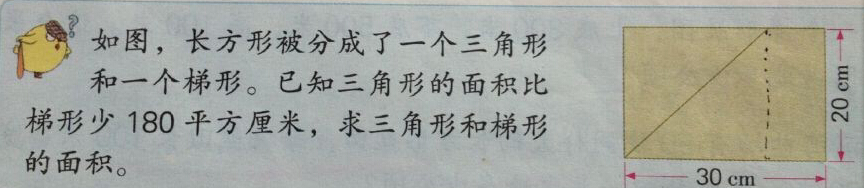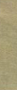1.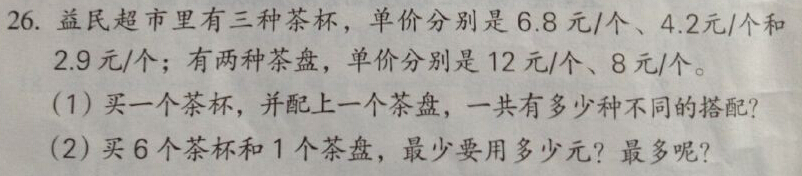2.求下面各个组合图形的面积。（单位：厘米）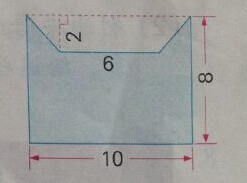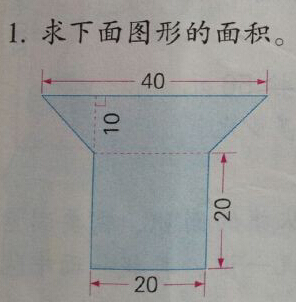3.求四边形ABCD的面积。（单位：厘米）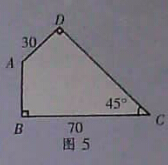4.一列快车和一列慢车相向而行，快车长270米，慢车长360米，坐在快车上的人看慢车驶过的时间是12秒，那么坐在慢车上的人看见快车驶过的时间是多少秒？

5.马小虎在计算25.2除以一个数时，不小心把除数的小数点向右多点了一位，结果得16.8.这道题目的除数是多少？